# PHY4: ELECTRO MAGNETISM

##### This unit is about Electro-magnetism and where it applies.

ELECTRO MAGNETISM

Magnetic flux

This refers to the number of magnetic field lines crossing a given area.

Magnetic effect of current

When an electric current flows in a wire a magnetic field is created around the wire .The field pattern created can be plotted by means of a plotting compass or iron fillings and the results shows that the magnetic field lines are concentric circles with the wire at the centre.y

The low of currents

Like current attract, unlike currents repel.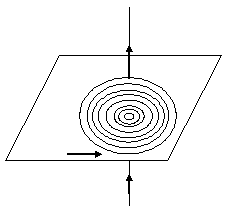The direction of the magnetic field depends on the direction of the current.

Direction of the current is given by the right hand grip or Fleming Maxwell rule. The rule station of current the direction of the thumb pointing in the direction of currents the direction of the other fingers gives the direction of the magnetic field or flux.

Magnetic field line due to a current flowing in a narrow circular coilThe direction of the magnetic field lines is always the same the centre of the coil.

Magnetic flux due to current in the solenoid

The direction of the magnetic field lines produced when a warrant flows through a solenoid is the same as that of a bar magnet one end acts as a north pole and the other as the South Pole;

When the coil is viewed from one and the current appears to be flowing in the clockwise direction that end of the solenoid is a south pole .If on the other hand the direction is clockwise the directions a north pole.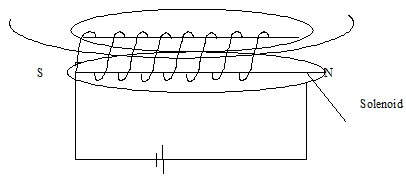The magnetic field of a solenoid is the same as that of a bar magnet.

ELECTRO MAGNETIC INDUCTION

This is the production of electricity from magnetism.

Faradays experiments on electromagnetic induction

The experiment uses a cylindrical coil of weapon wire would round a paper tube whose ends are connected to a sensitive galvanometer.

When a magnet is plunged into a coil its noted  that the galvanize   pointer gives  deflection showing  that  current  has  been  induced  in  the coil.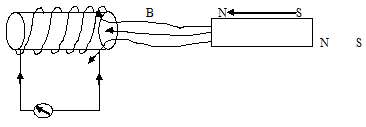Induced current makes and when the magnet is plunged Ba North Pole. Into the coil.

When the magnet is plunged out of the coil, the galvanometer gives another deflection this time in the opposite direction to the one before.When the magnet is plunged out of the coil, induced current makes end Ba south pole.

This effect of production of current is called electromagnet induction.

Note

No current is induced when there is no relative’s motion between the magnet and the coil hence on e.m.f is induced whenever there is a change in the magnetic flux linked with the coil.

The strength of the induced current depends on;-

-The number of turns in the coil.

– The strength of magnet.

-The speed with which the magnet is plunged in the coil.

A similar observation is obtained when two coils are placed side by side with one connected a coil and a switch while the other is connector a sensitive galvanometer.When a witch K is closed, induced current flows in one direction and the galvanometer gives a deflection in the opposite direction. This phenomenon is summarized into two laws called the laws of electromagnetic induction.

This states that whenever there is a change in the magnetic flux linked with a circuit on e.m.f is induced the strength of which is proportional to the rate of change of the flux linked with the circuit.

Lenz’s law;

This state that “The induced current always flows in such a direction as to oppose the change producing it.

Thus when the south poles of a magnet is plunged into the coil  the  induced  current  flows  in  such  a direction  that  the  end  near  to  the   magnet   behaves  like  south  pole  as  to  repel  the  incoming  south pole.  If  the  magnet  is  pulled  away, the  current  flows  in  such  away  as  to  make  the  end  near  the  magnet  north pole  so as to  attract  the  moving  away  south  pole.

Fleming right hard role

If the thumb finger the first finger and the second finger are held so that all the three are mutually perpendicular with the first finger pointing in the direction of the current, then the thumb finger points in the direction of motion.

ELECTROMAGNET

An electromagnet is bar of soft iron having coils of insulated copper wire, wound in opposite directions on the bar .The basis of an electromagnet is a soft iron rod.

An electromagnet is the basis of a soft iron rod with several turns of insulated wire wrapped around it.When a current is passed through the wire the soft iron get to magnetize and behaves like a magnet with one end having a north pole and the other a south pole. However when the current is switched off the soft iron loses the magnetism.

This property of soft iron is utilized whenever strong magnetism is required for a short time (temporarily).

On the other hand, when steel is used instead of soft iron a relatively weaker magnet is obtained. But a switching off the steel does not lose all its magnetism.

The strength of electromagnet can be increased by;

• Increasing the number of turns of the wire
• Increasing on the magnitude of current
• Using a soft iron

A strong electromagnet can be made by using a U-shaped soft iron core. This has an advantage that both poles may be used.

Uses of electromagnets

• Used in lifting magnets such magnets are used for transportation of very heavy machines ports with in a factory.The principal of the electromagnet can be used in making an electric bell.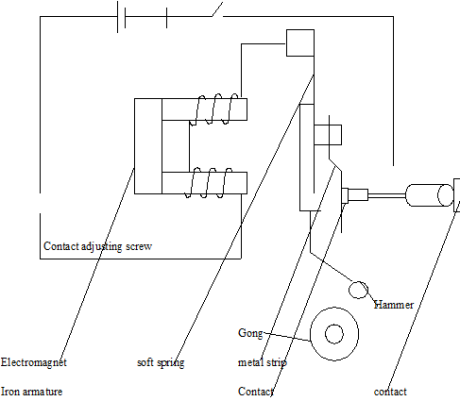Mode of operation

When the switch closed current flows in the coils of the electromagnet which becomes magnetized and attracts the soft iron armature. The hammer hits the gong and the circuit is broken at the armature. The sparingly metal strip is then able to pull the armature back, remarking contact and so completing the circuit again .This cycle is repeated and the bell rings continually.

THE A.C GENERATOR

Structure

The a.c generator consists of are rectangular PQRS coil between two poles of strong permanent magnet.

The coil is connected to two slip rings which are in sliding contact with carbon brushes to which a load is connected.Mode of operation

The rectangular coil PQRS is rotated in magnetic field provided by the concave pole pieces of a permanent magnet .The coil cuts the magnetic field lines (the magnetic field linked with the coil changed) and an e.m.g is induced in the coil. The direction of the induced e.m.g changes whenever the plane of the coil passes a position when it is perpendicular to the magnetic field. The induced e.m.g is load a way by the carbon brushes which are in slinging contact with the slip rings.

Note

The voltage generated by the generator varies with time from zero to maximum.

• Maximum output voltage is obtained when the coil is horizontal and the magnets are in a vertical plane or when the plane of the coil is parallel to the magnetic field .This is because the larger sides of the coil are cutting the magnetic field lines at the greatest rate.
• Zero output is obtained when the plane of the coil is horizontal and the magnetic field is when the plane of the coil is perpendicular to the magnetic field. At this point the loner sides of the coil do not cut the magnetic flux (field lines) and these sides are moving along the magnetic flux.

The magnitude of the induced e.m.f depends on the following factors;

-Number of turns in the coil.

-Speed of rotation of the coil.

-Area of the coil.

-Winding the coil on a soft iron armature so as to concentrate the magnetic flux.

Power losses in the generator are due to;

-Heat losses, I R due to resistance of the coil windings. This is minimized by using a coil made of low resistance copper wires.

-Eddy current losses (also inform of heat) .This is minimized by using a laminated iron core.

-Work done against friction in the bearings. Minimized by lubrication.

Venation of induced e.m.f with timeTHE D.C MOTOR

Construction

It consists of a rectangular coil PQRS between the poles of a permanent magnet .The coil is connected to a split ring commutate which is in sliding with carbon brushes .The carbon brushes are connected to a source of voltage.

When current is switched on by closing switch K side PS experience a down ward force while CB experiences an upward force. The two forces form a couple which rotates a coil until it is vertical. In this position, the brushes are then in line with the gaps in the split ring commentators, and the current stops.

Inertia causes the coil to overshoot its contact from one brush to another .This reverses the current through the coil and also the directions of the forces on its sides. The coil thus rotates in the some direction in this case anti-clockwise.

Calculations

If Vis the supply p.d applied to run the motor from the battery .In the armature current, as the motor runs an e.m.f called the back e.m.f Eb, is induced in the motor windings opposite direction to the applied p.d v.

(Power supply)  = (power developed in the motor) + (power loss)

LaV         =           La Eb          +  La2Ra where Ra is the resistance of the armature windings.

Or

La Eb = LaV -La 2Ra

Division through by La – Eb = V- La Ra

THE D.C GENERATOR / DYNAMO

It consists of a rectangular coil is PQRS between the pole of a permanent magnet .The coil connected to a split ring commuter which is in sliding contact with carbon brushes.

The carbons are connected to a load.When the coil is the magnetic field, the magnetic flux linked with it changes. An e.m.f is induced in the coil. The brushes are arranged so that when the coil is passing the vertical position, the two halves of the split ring one just on the point of changing contact from one brush to another.

Starting with the coil in the vertical position, the e.m.f generated during the first half rotation rises from zero to a maximum and then falls to zero again.

As for as the external resistance R is concerned, the right hand brush is positive and the left hand brush is negative. When the coil passes the vertical position, the correct in the coil changes as the brushes change contact but the polarities of the brush remain the same.

A graph showing the variation of induced e.m.f against time is as shown below.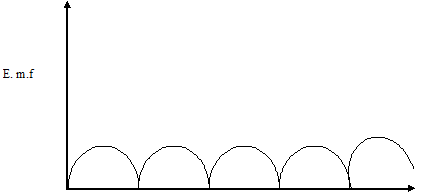THE TRANSFORMER

It is a device for stepping up or down, on alternating voltage .It works on the principle of mutual induction.Where Vp = primary voltage                                      Vs    = Secondary voltages

Np = Number of turns in the primary               Ns = Number of turns in the secondary.

A transformer consists of two coils, the primary coil and the secondary coil would on a soft iron core. The transformer works on the principle of mutual inductance.

When an alternating voltage is applied to a primary coil the resulting magnetic flux linked with the secondary change and an e.m.f is induced in the secondary the magnitude of the induced e.m.f Vs depends on the magnitude of the e.m.f Vs applied to the primary and the relative number of turns in the two coils.

If the number of turns Ns in the secondary coil exceed the number of turns Np in the primary ie if Ns Np the transformer is a stop up .

If the number of turns in the primary exceeds that in the secondary ie if Ns  Np the transformer is stop down.

For any transformer,Energy losses in a transformer

1 -Heating affect the core due to eddy current. This is minimized by using A laminated core.

2 -Magnetic flux leakage (not all the primary flux links the secondary).This is reduced by winding the secondary coil over the primary.

3 -loss of energy due to the continually changing direction of the molecular magnetism in the core. (Hysteresis loss).This induced by using a magnetically soft iron core.

4 -Energy loss due to resistance of the wires (coils) in form of heat (1 R).This is minimized by using low resistance copper wires.

Conversation of a millimeter to an ammeter

A millimeter is a device for measuring very small current (of order 10-3 A).It can be converted into an a ammeter cable of measuring large current (up 10A) by connecting a small current called a shunt in parallel to the main resistance of coil .The shunts serves the purpose of directing the most of the current in order to protect the coil.

Example

A millimeter has a coil resistance of 20 and bashful scale deflection for a current of 5mA.Explain how this millimeter can be converted into an ammeter which can measure a current up to 5A.

Solution

Rs = Resistance of the shunt, Is = current through the shuntThus a resistance of 0.02 should be connected in parallel to the main coil resistance in order to convert this millimeter of f.s.d 5mA to an ammeter measuring up to 5A.

Conversion of a millimeter in to a voltage

A millimeter used to measure up 5mA and having a coil resistance of 20 can also be used as a millimeter measuring up to (0.005×20)=0.1v .It can be converted into a voltmeter measuring p.d up to say 10v by connecting a resister called a multiplier.

The D.C motor (add)

The motor effects are where by a wire carrying a current in a magnetic field experiences a force. The direction of the force is determined by Flemings left hand rule (the motor rule).

### ASSIGNMENT : ELECTRO MAGNETISM assignmentMARKS : 10  DURATION : 1 week, 3 days

SEE ALL
•YOU